## A. Dynamic Framework

The dynamic framework of the model is based on the quasi-compressible system . In this framework, each variables is divided into horizontally uniform basic state and residual perturbation, and prognostic equations of residual perturbations are solved.

### Density, Exner function, and Virtual Potential temperature

Derivation of the momentum and pressure equations requires use of the equation of state that includes the effects of condensible species.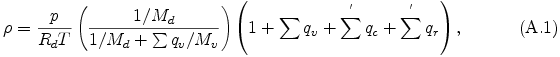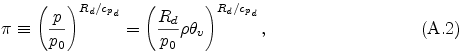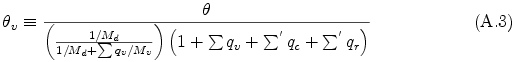and potential temperature θ is defined as θ = T / π.

### Momentum equations

The momentum equations are expressed in the following forms: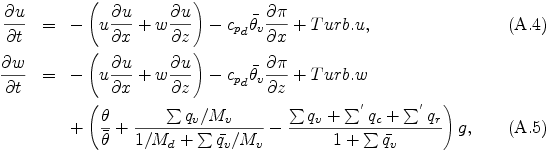where u is velocity in x direction, w is velocity in z direction, g is the acceleration due to gravity, and Turb.u and Turb.w refer to subgrid scale turbulent momentum mixing described in Appendix C. The variables denoted with over bar - represent horizontally uniform basic state.

### Pressure equation

The pressure equation is derived by using the compressible continuity equation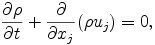eq. (A.1)--(A.3) , and the thermodynamic equation (A.7), which will be described later.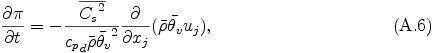### Thermodynamic equation

The thermodynamic equation can be written in the form: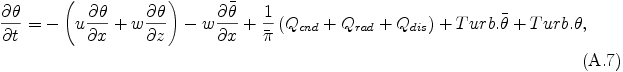where Qcnd refers to heating by condensation described in Appendix B, Qrad refers to radiation heating, and Qdis and Turb.θ refer to dissipative heating and subgrid scale turbulent mixing of potential temperature which are described in Appendix C, respectively.

### Conservation equations for condensible species

The conservation equations for condensible species can be written in the form: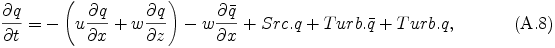where Src.q refers to source terms described in Appendix B and Turb.q refers to subgrid scale turbulent mixing terms described in Appendix C.